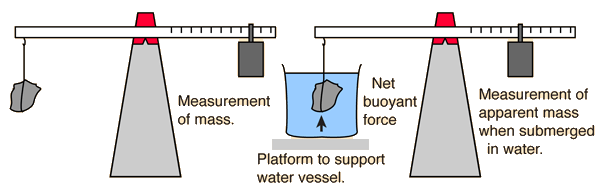# Archimedes' PrincipleArchimedes' principle aids in the experimental determination of density by providing a convenient and accurate method for determining the volume of an irregularly shaped object, like a rock.

If an object is massed in air and found to have mass m = grams

and is then submerged in water and found to have apparent mass m' = grams

then it has displaced m - m' = grams of water. Since water has a density of 1 gram/cm3, this implies

Volume of object = V = cm3.
 The density of the object is then ρ = m/V = gm/cm3.

 How many digits are significant?
Note that in the experimental arrangement, the container of water in which the object is submerged is supported on a platform so that the balance scale does not include the measurement of the mass of the water and its container.

### Density and buoyancy in a general fluid

Index

Buoyancy concepts

 HyperPhysics***** Mechanics ***** Fluids R Nave
Go Back